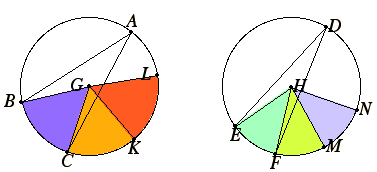# Proposition 33

Angles in equal circles have the same ratio as the circumferences on which they stand whether they stand at the centers or at the circumferences.

Let ABC and DEF be equal circles, and let the angles BGC and EHF be angles at their centers G and H, and the angles BAC and EDF angles at the circumferences.

I say that the circumference BC is to the circumference EF as the angle BGC is to the angle EHF, and as the angle BAC is to the angle EDF.Make any number of consecutive circumferences CK and KL equal to the circumference BC, and any number of consecutive circumferences FM, MN equal to the circumference EF, and join GK and GL and HM and HN.

III.27

Then, since the circumferences BC, CK, and KL equal one another, the angles BGC, CGK, and KGL also equal one another. Therefore, whatever multiple the circumference BL is of BC, the angle BGL is also that multiple of the angle BGC.

For the same reason, whatever multiple the circumference NE is of EF, the angle NHE is also that multiple of the angle EHF.

III.27

If the circumference BL equals the circumference EN, then the angle BGL also equals the angle EHN; if the circumference BL is greater than the circumference EN, then the angle BGL is also greater than the angle EHN; and, if less, less.

There being then four magnitudes, two circumferences BC and EF, and two angles BGC and EHF, there have been taken, of the circumference BC and the angle BGC equimultiples, namely the circumference BL and the angle BGL, and of the circumference EF and the angle EHF equimultiples, namely the circumference EN and the angle EHN.

And it has been proved that, if the circumference BL is in excess of the circumference EN, the angle BGL is also in excess of the angle EHN; if equal, equal; and if less, less.

V.Def.5

Therefore the circumference BC is to EF as the angle BGC is to the angle EHF.

But the angle BGC is to the angle EHF as the angle BAC is to the angle EDF, for they are doubles respectively.

Therefore also the circumference BC is to the circumference EF as the angle BGC is to the angle EHF, and the angle BAC to the angle EDF.

Therefore, angles in equal circles have the same ratio as the circumferences on which they stand whether they stand at the centers or at the circumferences.

Q.E.D.

## Guide

This proposition stands apart from the rest in this book since it depends on none of them. It is, however, analogous to the first proposition VI.1 of the book. Whereas VI.1 established a proportion between lines and plane figures, this proposition establishes a proportion between angles and portions of circumferences cut off by those angles.

In the Elements Euclid restricted his study of lengths of arcs to circles of the same radius. He did not compare arcs of different sized circles. Later, however, Archimedes did just that in his Measurement of a Circle.

This proposition is used in three consecutive propositions in Book XIII starting with XIII.8 to convert statements about arcs to statements about angles. Incidentally, all three have to do with regular pentagons inscribed in circles.